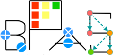Basic Polynomial Algebra Subprograms (BPAS)  v. 1.652
ComplexRationalNumber Class Reference

An arbitrary-precision complex rational number. More...

`#include <ComplexRationalNumber.hpp>`

Simplified semantic inheritance diagram for ComplexRationalNumber:Full inheritance diagram for ComplexRationalNumber:
[legend]

## Public Member Functions

ComplexRationalNumber (const mpq_class &_a, const mpq_class &_b=mpq_class(1))

ComplexRationalNumber (const ComplexRationalNumber &c)

ComplexRationalNumber (int _a, int _b=1, int _c=0, int _d=1)

ComplexRationalNumber (const Integer &c)

ComplexRationalNumber (const RationalNumber &c)

ComplexRationalNumber (const SmallPrimeField &c)

ComplexRationalNumber (const BigPrimeField &c)

ComplexRationalNumber (const GeneralizedFermatPrimeField &c)

ComplexRationalNumber (const DenseUnivariateIntegerPolynomial &c)

ComplexRationalNumber (const DenseUnivariateRationalPolynomial &c)

ComplexRationalNumber (const SparseUnivariatePolynomial< Integer > &c)

ComplexRationalNumber (const SparseUnivariatePolynomial< RationalNumber > &c)

ComplexRationalNumber (const SparseUnivariatePolynomial< ComplexRationalNumber > &c)

template<class Ring >
ComplexRationalNumber (const SparseUnivariatePolynomial< Ring > &c)

ComplexRationalNumberoperator= (const ComplexRationalNumber &c)
Copy assignment.

ComplexRationalNumberoperator= (const mpq_class &k)

ComplexRationalNumberoperator= (int k)

ComplexRationalNumbersetRealPart (const RationalNumber &r)

ComplexRationalNumbersetRealPart (const mpq_class &k)

ComplexRationalNumbersetRealPart (int k)

ComplexRationalNumbersetImaginaryPart (const RationalNumber &r)

ComplexRationalNumbersetImaginaryPart (const mpq_class &k)

ComplexRationalNumbersetImaginaryPart (int k)

ComplexRationalNumberset (const RationalNumber &ka, const RationalNumber &kb)

ComplexRationalNumberset (const mpq_class &ka, const mpq_class &kb)

ComplexRationalNumberset (const mpq_class &ka, int kb)

ComplexRationalNumberset (int ka, const mpq_class &kb)

ComplexRationalNumberset (int ka, int kb)

bool isZero () const
Is a zero. More...

void zero ()
Assign to zero. More...

bool isOne () const
Is a 1. More...

void one ()
Assign to one. More...

bool isNegativeOne () const
Is a -1. More...

void negativeOne ()
Assign to negative one. More...

int isConstant () const
Is a constant. More...

ComplexRationalNumber unitCanonical (ComplexRationalNumber *u=NULL, ComplexRationalNumber *v=NULL) const
Obtain the unit normal (a.k.a canonical associate) of an element. More...

bool operator== (const ComplexRationalNumber &c) const
Equality test,. More...

bool operator== (const mpq_class &k) const

bool operator== (int k) const

bool operator!= (const ComplexRationalNumber &c) const
Inequality test,. More...

bool operator!= (const mpq_class &k) const

bool operator!= (int k) const

ComplexRationalNumber operator+ (const ComplexRationalNumber &c) const
Addition.

ComplexRationalNumberoperator+= (const ComplexRationalNumber &c)
Addition assignment.

ComplexRationalNumber operator- (const ComplexRationalNumber &c) const
Subtraction.

ComplexRationalNumberoperator-= (const ComplexRationalNumber &c)
Subtraction assignment.

ComplexRationalNumber operator- () const
Negation.

ComplexRationalNumber operator* (const ComplexRationalNumber &c) const
Multiplication.

ComplexRationalNumberoperator*= (const ComplexRationalNumber &c)
Multiplication assignment.

ComplexRationalNumberoperator*= (const mpq_class &c)

ComplexRationalNumberoperator*= (int c)

ComplexRationalNumber operator^ (long long int e) const
Overload operator ^ replace xor operation by exponentiation. More...

ComplexRationalNumberoperator^= (long long int e)
Exponentiation assignment.

ExpressionTree convertToExpressionTree () const
Convert this to an expression tree. More...

ComplexRationalNumber operator/ (const ComplexRationalNumber &c) const
Exact division.

ComplexRationalNumberoperator/= (const ComplexRationalNumber &c)
Exact division assignment.

ComplexRationalNumber operator% (const ComplexRationalNumber &c) const
Get the remainder of *this and b;.

ComplexRationalNumberoperator%= (const ComplexRationalNumber &c)
Assign *this to be the remainder of *this and b.

ComplexRationalNumber gcd (const ComplexRationalNumber &c) const
GCD(a, b) More...

Factors< ComplexRationalNumbersquareFree () const
Compute squarefree factorization of *this.

ComplexRationalNumber euclideanSize () const
Get the euclidean size of *this.

ComplexRationalNumber euclideanDivision (const ComplexRationalNumber &b, ComplexRationalNumber *q=NULL) const
Perform the eucldiean division of *this and b. More...

ComplexRationalNumber extendedEuclidean (const ComplexRationalNumber &b, ComplexRationalNumber *s=NULL, ComplexRationalNumber *t=NULL) const
Perform the extended euclidean division on *this and b. More...

ComplexRationalNumber quotient (const ComplexRationalNumber &b) const
Get the quotient of *this and b.

ComplexRationalNumber remainder (const ComplexRationalNumber &b) const
Get the remainder of *this and b.

ComplexRationalNumber inverse () const
Get the inverse of *this.

RationalNumber realPart () const

RationalNumber imaginaryPart () const

ComplexRationalNumber conjugate () const

void print (std::ostream &out) const
Print the Ring element. More...

## Static Public Attributes

static mpz_class characteristic

static RingProperties properties

## Detailed Description

An arbitrary-precision complex rational number.

## ◆ convertToExpressionTree()

 ExpressionTree ComplexRationalNumber::convertToExpressionTree ( ) const
inlinevirtual

Convert this to an expression tree.

returns an expression tree describing *this.

Implements ExpressionTreeConvert.

## ◆ euclideanDivision()

 ComplexRationalNumber ComplexRationalNumber::euclideanDivision ( const ComplexRationalNumber & b, ComplexRationalNumber * q = `NULL` ) const
virtual

Perform the eucldiean division of *this and b.

Returns the remainder. If q is not NULL, then returns the quotient in q.

Implements BPASEuclideanDomain< ComplexRationalNumber >.

## ◆ extendedEuclidean()

 ComplexRationalNumber ComplexRationalNumber::extendedEuclidean ( const ComplexRationalNumber & b, ComplexRationalNumber * s = `NULL`, ComplexRationalNumber * t = `NULL` ) const
virtual

Perform the extended euclidean division on *this and b.

Returns the GCD. If s and t are not NULL, returns the bezout coefficients in them.

Implements BPASEuclideanDomain< ComplexRationalNumber >.

## ◆ gcd()

 ComplexRationalNumber ComplexRationalNumber::gcd ( const ComplexRationalNumber & c ) const
inlinevirtual

GCD(a, b)

Parameters
 b The other rational number

Implements BPASGCDDomain< ComplexRationalNumber >.

## ◆ isConstant()

 int ComplexRationalNumber::isConstant ( ) const
inline

Is a constant.

Parameters

## ◆ isNegativeOne()

 bool ComplexRationalNumber::isNegativeOne ( ) const
inline

Is a -1.

Parameters

## ◆ isOne()

 bool ComplexRationalNumber::isOne ( ) const
inlinevirtual

Is a 1.

Parameters

Implements BPASRing< ComplexRationalNumber >.

## ◆ isZero()

 bool ComplexRationalNumber::isZero ( ) const
inlinevirtual

Is a zero.

Parameters

Implements BPASRing< ComplexRationalNumber >.

## ◆ negativeOne()

 void ComplexRationalNumber::negativeOne ( )
inline

Assign to negative one.

Parameters

## ◆ one()

 void ComplexRationalNumber::one ( )
inlinevirtual

Assign to one.

Parameters

Implements BPASRing< ComplexRationalNumber >.

## ◆ operator!=()

 bool ComplexRationalNumber::operator!= ( const ComplexRationalNumber & ) const
inlinevirtual

Inequality test,.

returns true iff not equal.

Implements BPASRing< ComplexRationalNumber >.

## ◆ operator==()

 bool ComplexRationalNumber::operator== ( const ComplexRationalNumber & ) const
inlinevirtual

Equality test,.

returns true iff equal

Implements BPASRing< ComplexRationalNumber >.

## ◆ operator^()

 ComplexRationalNumber ComplexRationalNumber::operator^ ( long long int e ) const
inlinevirtual

Overload operator ^ replace xor operation by exponentiation.

Parameters
 e The exponentiation

Implements BPASRing< ComplexRationalNumber >.

## ◆ print()

 void ComplexRationalNumber::print ( std::ostream & ostream ) const
virtual

Print the Ring element.

Derived classes may override this to get custom printing that may be more expressive (and prettier) than expression tree printing.

Reimplemented from BPASRing< ComplexRationalNumber >.

## ◆ unitCanonical()

 ComplexRationalNumber ComplexRationalNumber::unitCanonical ( ComplexRationalNumber * u = `NULL`, ComplexRationalNumber * v = `NULL` ) const
virtual

Obtain the unit normal (a.k.a canonical associate) of an element.

If either parameters u, v, are non-NULL then the units are returned such that b = ua, v = u^-1. Where b is the unit normal of a, and is the returned value.

Implements BPASRing< ComplexRationalNumber >.

## ◆ zero()

 void ComplexRationalNumber::zero ( )
inlinevirtual

Assign to zero.

Parameters

Implements BPASRing< ComplexRationalNumber >.

The documentation for this class was generated from the following file: